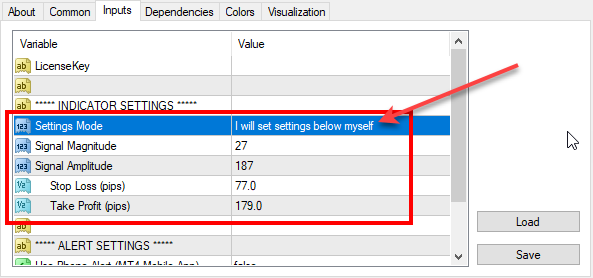# Re-optimized FxMagnetic indicator on EURUSD (2018-09-17)

We’ve reoptimized FxMagnetic indicator settings on EURUSD. Here are the latest parameters for all EURUSD timeframes that you should use for best MT4 indicator performance.To set parameters manually you need to switch the Settings Mode to: I will set settings below myself

Don’t have FxMagnetic indicator yet? You can buy FxMagnetic here.

## 1-minute chart:

Signal Magnitude = 100

Signal Amplitude = -200

Stop Loss (pips) = 40

Take Profit (pips) = 50

## 5-minute chart:

Signal Magnitude = 20

Signal Amplitude = -124

Stop Loss (pips) = 60

Take Profit (pips) = 110

## 15-minute chart:

Signal Magnitude = 88;

Signal Amplitude = -213;

Stop Loss (pips) = 60;

Take Profit (pips) = 102;

## 30-minute chart:

Signal Magnitude = 44;

Signal Amplitude = -200;

Stop Loss (pips) = 55;

Take Profit (pips) = 100;

## 1-hour chart:

Signal Magnitude = 22;

Signal Amplitude = -187;

Stop Loss (pips) = 57;

Take Profit (pips) = 91;

## 4-hour chart:

Signal Magnitude = 40;

Signal Amplitude = -140;

Stop Loss (pips) = 109;

Take Profit (pips) = 186;

## Daily chart:

Signal Magnitude = 100;

Signal Amplitude = -40;

Stop Loss (pips) = 300;

Take Profit (pips) = 480;

## Weekly chart:

Signal Magnitude = 20;

Ind0Param1 = -40;

Stop Loss (pips) = 360;

Take Profit (pips) = 1000;

## Monthly chart:

Signal Magnitude = 20;

Signal Amplitude = -80;

Stop Loss (pips) = 360;

Take Profit (pips) = 1000;